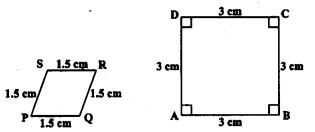# KSEEB SSLC Class 10 Maths Solutions Chapter 2 Triangles Ex 2.1

KSEEB SSLC Class 10 Maths Solutions Chapter 2 Triangles Ex 2.1 are part of KSEEB SSLC Class 10 Maths Solutions. Here we have given Karnataka SSLC Class 10 Maths Solutions Chapter 2 Triangles Exercise 2.1.

## Karnataka SSLC Class 10 Maths Solutions Chapter 2 Triangles Exercise 2.1

Question 1.
Fill In the b1ank using the correct word given in brackets:
i) All circles are _______ . (congruent, similar)
Solution:
congruent

ii) All squares are _______. (similar, congruent)
Solution:
similar

iii) All ______ triangles are similar. (isosceles, equilateral)
Solution:
equilateral

a

iv) Two polygons of the same number of sides are similar, if
(a) their corresponding angles are _______ and
(b) their corresponding sides are _______. (equal, proportional).
Solution:
(a) equal
(b) proportional

Question 2.
Give two different examples of pair of
i) similar figures
Solution:
coin, wheel of a cart.

ii) non-similar figures.
Solution:
A square Rhombus

Question 3.
State whether the following quadrilaterals are similar or not:Solution:
Here, two diagrams PQRS and ABCD are rhombus and square whose sides are in proportional. But the corresponding angle is not equal. Hence these are not similar.

We hope the given KSEEB SSLC Class 10 Maths Solutions Chapter 2 Triangles Ex 2.1 will help you. If you have any query regarding Karnataka SSLC Class 10 Maths Solutions Chapter 2 Triangles Exercise 2.1, drop a comment below and we will get back to you at the earliest.

a